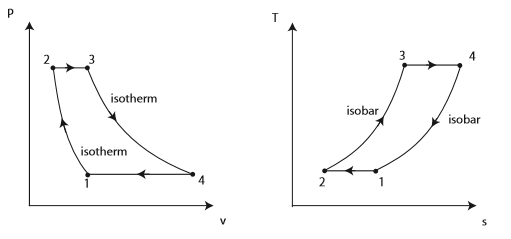##### Question

In: Mechanical Engineering

# ericsson cycle

Consider an ideal Ericsson cycle with air as the working ﬂuid executed in a steady-ﬂow system. Air is at a temperature of 270 degrees and a pressure of  120 kPa at the beginning of the isothermal compression process, during which 150 kJ/kg of heat is rejected. Heat transfer to air occurs at a temperature of 1200K. Determine

(a) the maximum pressure in the cycle

(b) the network output per unit mass of air

(c) the thermal eﬃciency of the cycle

## Solutions

##### Expert SolutionAn Ericsson cycle involves an isothermal heat-addition process and an isothermal heat-rejection process. the Ericsson cycle diﬀer from the Carnot cycle in that the two isentropic processes in the Carnot cycle are replaced by two constant-pressure regeneration processes. The cycle utilizes regeneration, a process during which heat is transferred to a thermal energy storage device called a regenerator during one part of the cycle and is transferred back to the working ﬂuid during another part of the cycle.

the p-v and T-s diagrams are shown in the diagram above and the processes are as follows:

1 → 2: isothermal compression,

2 → 3: isobaric heating in the combustion chamber,

3 → 4: isothermal expansion in the turbine,

4 → 1: isobaric heat transfer in surroundings

(A). The maximum pressure

the change in entropy can be given by:

S4-S3=qout/TL: (-150/300K

=-0.5KJ/Kg/K

The change of entropy can also be given by:

cp In(T4/T3) - R In(p4/p3)

from the T-s diagram, T3=T4=TL

the change in entropy can therefore be given by:

-0.5KJ/KG/K = Cp In1-(0.287kJ/KG/K) In( P4/P3)

-0.5KJ/KG/K = 0 - (0.287KJ/KG/K) In (P4/120Kpa)

p4 = 685.12Kpa which is our maximum pressure

(B)The net work output

Wnet = qin - qout

for an ideal reversible cycle, (qin/qout)=(TH/TL)

qin = qoutX(TH/TL)

= 150X(1200/300) = 600KJ/Kg

The net work is given by:

qin-qout

600KJ/kg - 150KJ/kg

Wnet = 450KJ/Kg

(c) The thermal efficiency of the cycle

thermal efficiency = 1 - (TL/TH)

= 1 - (300/1200)

0.75 = 75%

(a). maximum pressure = 685.12 Kpa

(b.). the net work output = 450kJ/Kg

(c). the thermal efficiency = 75%

## Related Solutions

##### LABELING THE CELL CYCLE DIAGRAM?
Label the cell cycle diagram using the discussion about cell cycle.
##### Which of these is NOT a product of the citric acid cycle?
Which of these is NOT a product of the citric acid cycle? CO2 FADH2 NADH + H+ acetyl CoA ATP
##### Which of these enters the citric acid cycle?
Which of these enters the citric acid cycle?  G3P glucose NADH + H+ acetyl CoA pyruvate
##### In general, what is the purpose of a checkpoint in the cell cycle?
In general, what is the purpose of a checkpoint in the cell cycle?
##### In an engine cycle like the one shown in Figure
In an engine cycle like the one shown in Figure    heat is added over a range of temperatures. Explain why even if it were run reversibly, its efficiency would be less than the Carnot limit defined by the high- and low-temperature set points and shown in the figure ( ηC = T4-T2)/T4. Is this in itself a disadvantage of such a cycle?
##### Which of the following accounts is not closed at the end of an accounting cycle? Multiple...
Which of the following accounts is not closed at the end of an accounting cycle? Multiple Choice A. Revenues B. Retained earnings C. Dividends D. Expenses
##### explain in what ways is unemployment impacted by the phases of the business cycle?
explain in what ways is unemployment impacted by the phases of the business cycle?
##### ifthe us is in the expansion phase of the business cycle, recoveringfrom a recession,...
if the us is in the expansion phase of the business cycle, recovering from a recession, which of the following variables is increasing?a. real gdpb.aggregate real incomec. cyclical unemploymentd. the natural rate of unemploymente. both a and b
##### There are different economic explanations or theories for the business cycle that seek explaining how the...
There are different economic explanations or theories for the business cycle that seek explaining how the economy’s output level, average price level, and unemployment / employment rates change together or co-vary. Explain in 200 to 300 words why economists seek these explanations and why these explanations when adopted by others for their use in business planning or legislating macroeconomic policy is important to you in your personal and working lives. Note there does not exist to date a single macroeconomic...